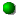# \$B%+%?%j%J(B-\$B%j%K%"WB@1(B

227P/Catalina-LINEAR###\$B2s5"5-O?(B\$BH/8+(B\$B=P8=(B\$B4QB,\$5\$l\$J\$+\$C\$?2s5"(B\$BH/8+A0\$N=P8=(B\$BH/8+A0\$N4QB,\$5\$l\$J\$+\$C\$?2s5"(B\$B>-Mh\$N2s5"(B2024/03/08227P 2017/06/22227P/2009 S4 2010/09/03227P/2004 EW38 2003/11/19227P 1997/01/30##### 1997\$BG/\$N=P8=\$O(BMaik Meyer\$B;a\$N;XE&\$K\$h\$k\$b\$N(B(NK 1852)\$B!#(B 1997\$BG/6aF|E@DL2aF|\$O:4F#M55W;a\$N7W;;\$K\$h\$k\$b\$N!#(B